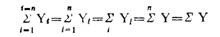# Arithmetic Mean: Characteristics, Applications and Limitations

The arithmetic mean,’ mean or average is calculated by summ­ing all the individual observations or items of a sample and divid­ing this sum by the number of items in the sample. For example, as the result of a gas analysis in a respirometer an investigator obtains the following four readings of oxygen percentages:

14.9

10.8

12.3

23.3

___________

Sum=61.3

He calculates the mean oxygen percentage as the sum of the four items divided by the number of items—here, by four. Thus the average oxygen percentage is

Mean = 61.3 / 4 =15.325%

Calculating a mean presents us with the opportunity for learning statistical symbolism. An individual observation is symbo­lized by Yi, which stands for the ith observation in the sample. Four observations could be written symbolically as Yi, Y2, Y3, Y4.

We shall define n, the sample size, as the number of items in a sample. In this particular instance, the sample size n is 4. Thus, in a large sample, we can symbolize the array from the first to the nth item as follows: Y1, Y2…, Yn. When we wish to sum items, we use the following notation:The capital Greek sigma, Ʃ, simply means the sum of items indica­ted. The i = 1 means that the items should be summed, starting with the first one, and ending with the nth one as indicated by the i = n above the Ʃ. The subscript and superscript are necessary to indicate how many items should be summed. Below are seen increasing simplifications of the complete notation shown at the extreme left:#### Properties of Arithmetic Mean:

1. The sum of deviations of the items from the arithmetic mean is always zero i.e.

∑(X–X) =0.

1. The Sum of the squared deviations of the items from A.M. is minimum, which is less than the sum of the squared deviations of the items from any other values.
2. If each item in the series is replaced by the mean, then the sum of these substitutions will be equal to the sum of the individual items.

#### Merits of A.M:

1. It is simple to understand and easy to calculate.
2. It is affected by the value of every item in the series.
3. It is rigidly defined.
4. It is capable of further algebraic treatment.
5. It is calculated value and not based on the position in the series.

#### Demerits of A.M:

1. It is affected by extreme items i.e., very small and very large items.
2. It can hardly be located by inspection.
3. In some cases A.M. does not represent the actual item. For example, average patients admitted in a hospital is 10.7 per day.
4. A.M. is not suitable in extremely asymmetrical distributions.

## One thought on “Arithmetic Mean: Characteristics, Applications and Limitations”

error: Content is protected !!